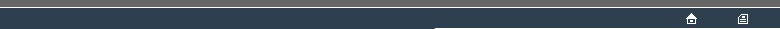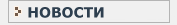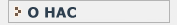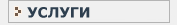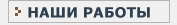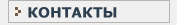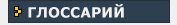СТАТИСТИЧЕСКИЙ В данном разделе Вы найдете все интересующие Вас термины и определения, связанные с статистикой и статистическими исследованиями.КЛИНИЧЕСКИЕ ИССЛЕДОВАНИЯ Необходимый перечень терминов и определений, используемых при проведении клинических испытанийP P-VALUE The ALPHA value arising from a statistical test. Also see : EXACT TEST(2) PAS2C One of a number of PROGRAMs for undertaking translations between STANDARD PROGRAMMING LANGUAGES. PASCAL [Named after the mathematician Blaise Pascal ( - )]. A PROGRAMMING LANGUAGE designed for clarity of expression when published in human-legible form, and for the teaching of programming. PASCAL is to some extent specialised for numerical work. A development is EXTENDED PASCAL. COMPILERS for PASCAL are widespread. PASCAL and EXTENDED PASCAL are each represented as STANDARD PROGRAMMING LANGUAGEs approved by ANSI and ISO. PERMUTATION This term has a distinct mathematical definition, but is also commonly used as a synonym for RE-RANDOMISATION. PERMUTATION TEST See : PERMUTATION, PITMAN PERMUTATION TEST(1), PITMAN PERMUTATION TEST(2). PITMAN PERMUTATION TEST(1) [Named after the statistician E.J. Pitman who described this test, and the PITMAN PERMUTATION TEST(2), in 1937; this is one of the earliest instances of an EXACT TEST(1)] An EXACT RE-RANDOMISATION TEST in which the TEST STATISTIC is the DIFFERENCE OF MEANS of two samples of univariate INTERVAL-SCALE data. . Also see : EQUIVALENT TEST STATISTIC, PITMAN PERMUTATION TEST(2). PITMAN PERMUTATION TEST(2) An EXACT RE-RANDOMISATION TEST in which the TEST STATISTIC is the MEAN DIFFERENCE of a single sample of univariate data measured under two circumstances as REPEATED MEASURES. Also see : PITMAN PERMUTATION TEST(1) POISSON DISTRIBUTION The distribution of number of events in a given time, arising from a POISSON PROCESS. This differs from the BINOMIAL DISTRIBUTION in that there is no upper limit, corresponding to the parameter 'n' of a BINOMIAL PROCESS, to the number of events which may occur. Also see : ODDS RATIO. POISSON PROCESS A process whereby events occur independently in some continuum (in many applications, time), such that the overall density (rate) is statistically constant but that it is impossible to improve any prediction of the position (time) of the next event by reference to the detail of any number of preceding observations. The corresponding distribution of intervals between events is an exponential distribution. The conventional example of a POISSON PROCESSES is concerned with occurence of radioactive emissions in a substantial sample of radioactive with a half-life very much longer than the total observation period. Also see : POISSON DISTRIBUTION. POPULATION A definable set of individual units to which the findings from statistical examination of a SAMPLE subset are intended to be applied. The POPULATION will generally much outnumber the SAMPLE. In RE-RANDOMISATION STATISTICs the process of applying inferences based upon the SAMPLE to the POPULATION is essentially informal. Also see : REPRESENTATIVE. POWER This is the probability that a statistical test will detect a defined pattern in data and declare the extent of the pattern as showing STATISTICAL SIGNIFICANCE. POWER is related to TYPE-2 ERROR by the simple formula : POWER = (1-BETA) ; the motive for this re-definition is so that an increase in value for POWER shall represent improvement of performance of a STATISTICAL TEST. For more detail, see : BETA. PROGRAM A sequence of instructions expressed in some PROGRAMMING LANGUAGE. Also see ALGORITHM(2). PROGRAMMABLE The characteristic of a COMPUTER which enables it to be used to undertake a variety of different processes on different occasions. Also see : ALGORITHM(2), PROGRAM, PROGRAMMING LANGUAGE, STANDARD PROGRAMMING LANGUAGE. PROGRAMMING LANGUAGE A formal code for expressing to a COMPUTER how a certain process should be undertaken. The translation from the code of the PROGRAMMING LANGUAGE to the OBJECT CODE of the appropriate COMPUTER is itself undertaken by a PROGRAM for that COMPUTER; the translation program may take the form of either a COMPILER of an INTERPRETER. Also see : ALGORITHM(1), ALGORITHM(2), PROGRAM. STANDARD PROGRAMMING LANGUAGES. PSEUDO-RANDOM A source of data which is effectively unpredictable although generated by a determinate process. Successive PSEUDO-RANDOM data are produced by a fixed calculation process acting upon preceding data from the PSEUDO-RANDOM sequence. To start the sequence it is necessary to decide arbitrarily upon a first datum, which is termed the SEED value. Also see : BOOTSTRAP, MONTE-CARLO TEST.P
P-VALUE
PAS2C
PASCAL
PERMUTATION
PERMUTATION TEST
PITMAN PERMUTATION TEST(1)
PITMAN PERMUTATION TEST(2)
POISSON DISTRIBUTION
POISSON PROCESS
POPULATION
POWER
PROGRAM
PROGRAMMABLE
PROGRAMMING LANGUAGE
PSEUDO-RANDOMНовости   О нас   Услуги   Наши работы   Статьи   Контакты   Глоссарий
Статистическая помощь! © 2005 - 2022 • Защита авторских прав